# Closed Loop Control of BLDC Motor using MATLAB simulink

Closed Loop Control of BLDC Motor: Closed loop control of brushless direct current (BLDC) motor is a system that is used for controlling the speed of BLDC motor. The BLDC motor is almost similar with brushed dc motor such as stepper motor. The only difference between BLDC motor and brushed dc motor is that we can easily control the speed of BLDC motor but we can’t control the angular position of this motor. Instead of this, we can easily control the angular position of brushed dc motor. Beside this, the BLDC motor has high power density and high torque to weight ratio as compared to stepper and induction motor. In this article we will learn the user how they can control the speed of BLDC motor by using PID (proportional integral derivative) controller with the help MATLAB Simulink. Beside this, we will also learn the user how they can make a new Simulink model in MATLAB. For making a new Simulink model first they will open the MATLAB software and then click on Simulink library browser then a new page would be opened shown is figure 1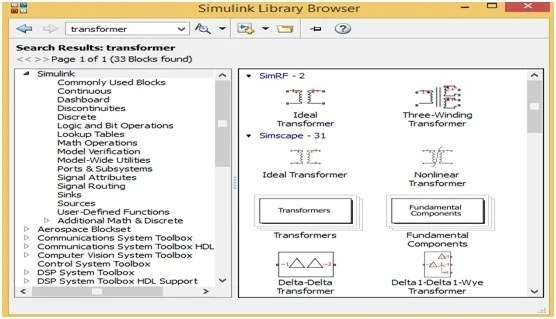Figure 1 MATLAB Simulink Library Browser

This MATLAB Simulink library browser have different options on their Manu bar as well as this page also have different components blocks. But for making any new circuit or model, first the user will click on new model option then a new blank page would be opened shown in figure 2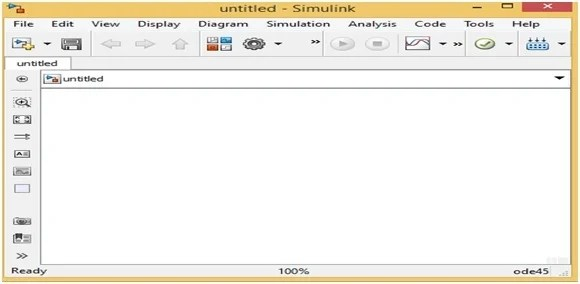Figure 2 MATLAB Simulink New Model

On this new blank page, the user can make any type of related circuit. For making any circuit the user will first choose or search the related components blocks. Here we want to make the closed loop control of BLDC motor circuit therefore we will have to search or choose the power electronics components blocks. For searching power electronics components blocks the user just write the word power electronic on search Manu bar then a new page would be opened which have all the power electronics components blocks such as power diode, MOSFET and thyristors etc. Shown in figure 3Figure 3 Power Electronics Components Blocks

Now the user can drag or place these components block on a new model page. For placing these block on a new model page just right click on any specific block and then click on option add this block on new model then this block will automatically place on a new model page. Similarly, the user can search PID controller block by writing the word PI on search Manu bar. When he writes the PI on search Manu bar then a new power controller page would be opened shown in figure 4.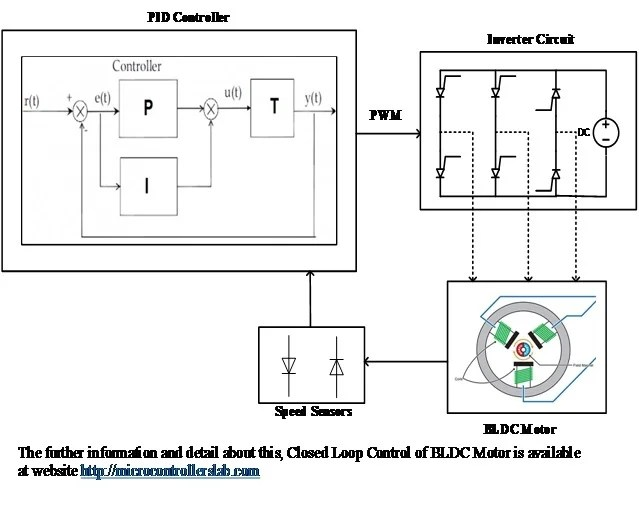Figure 4 PID Controller Blocks

By following the above step by step instructions, the user can easily make this closed loop control system for BLDC motor. Here we will not make this circuit on MATLAB Simulink page but the user can make this closed loop control system on MATLAB Simulink page after understanding the below block diagram of closed loop control of BLDC motor shown in figure 5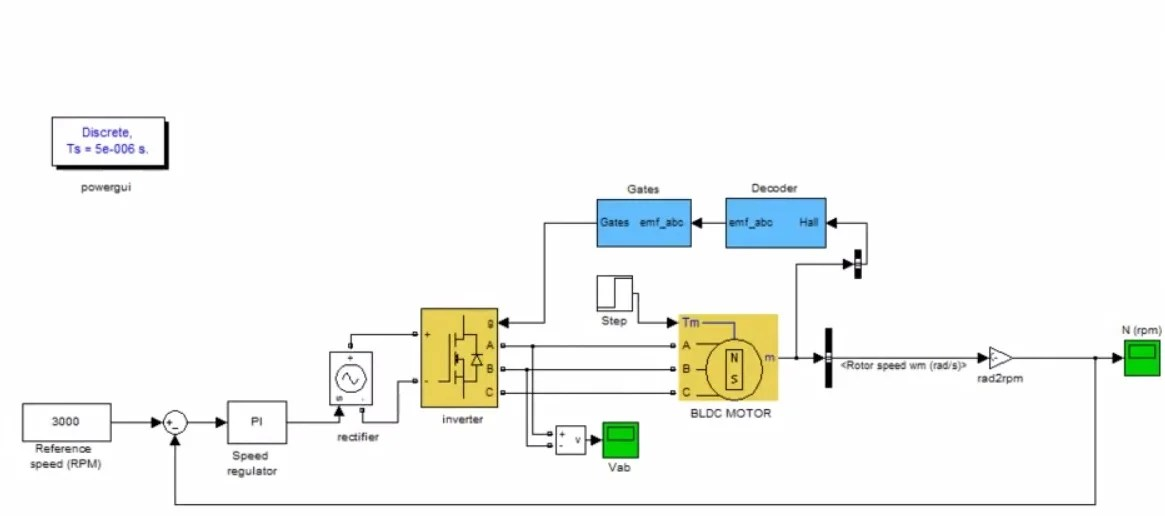Figure 5 Block of Closed Loop Control of BLDC Motor

In this closed loop control of BLDC motor system, the speed of dc motor is controlled through PID controller. For making this circuit the user will first make this circuit according to above block diagram and then tune PID controller according to their speed requirements. After tuning this PID controller now the controller is ready to give PWM to 6 thyristors. By changing the duty cycle of PWM pulse the speed of BLDC motor could be changed. The duty cycle would be changed through PID controller. The user can also attach the speed sensor with BLDC motor for checking the speed of motor and then for giving the feedback to PID controller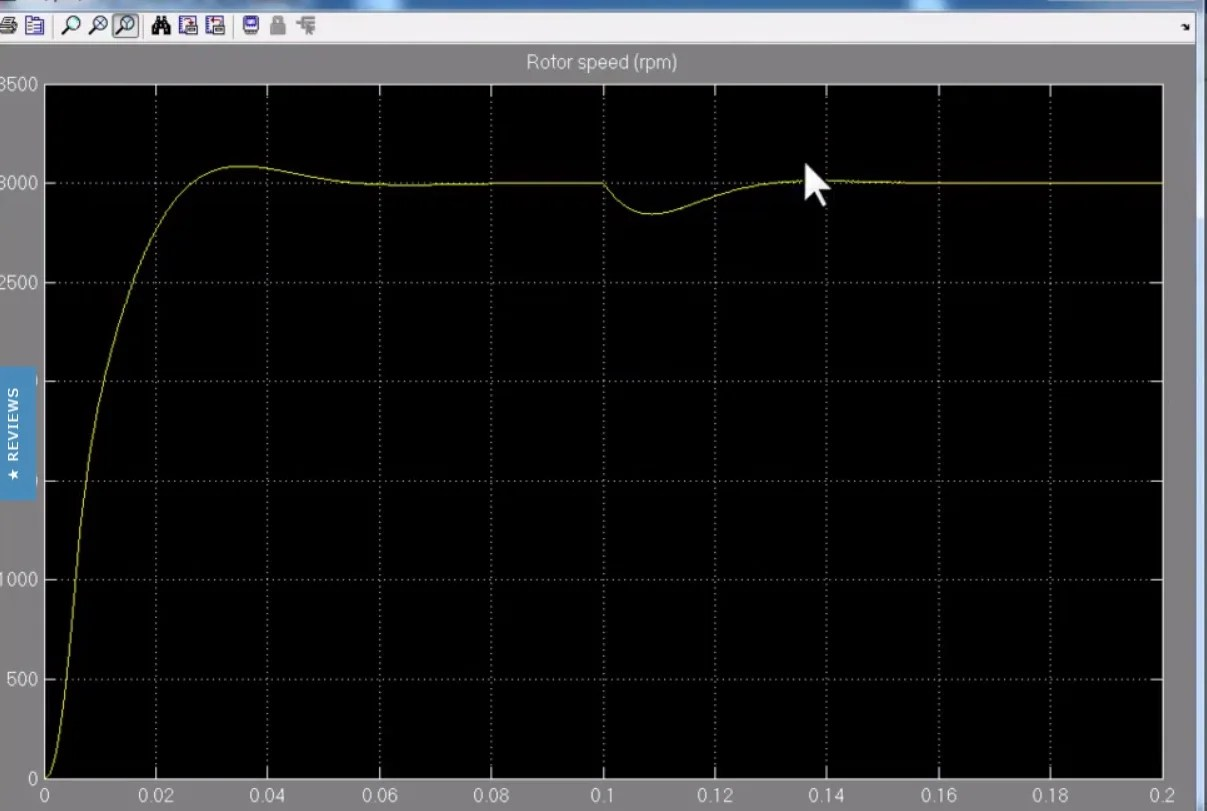### 2 thoughts on “Closed Loop Control of BLDC Motor using MATLAB simulink”

1. Hi,
This is a great article, but it seems incomplete.